Disclosure: This post may contain affiliate links, meaning when you click the links and make a purchase, we receive a commission.

# Percentage Difference Between Two Numbers in Excel (Using Formula)

In this article, we will see the formula to calculate the percentage difference between two Numbers in Excel. There is basically one formula to calculate this which is, % of Difference = (New Value-Old Value)/Old Value. Normally after applying this formula, we multiply the result with 100 to get the percentage of difference. But in Excel, you don’t need to multiply 100 with the resultant number. There is a built-in feature available in Excel which automatically formats a number into a percentage.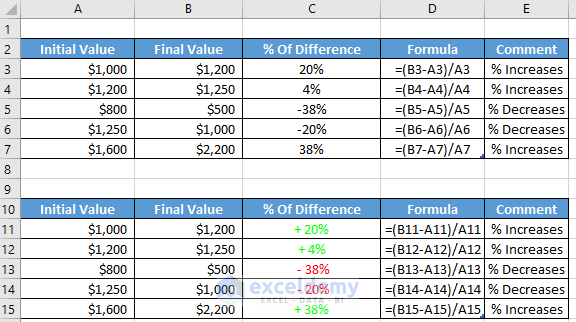## Calculate Percentage Difference Between Two Numbers

As I stated, the formula to calculate the percentage of difference is, % of Difference = (New Value-Old Value)/Old Value. After applying this formula, you will get your result in number format. So, you have to format it into a percentage to get the actual result. This formula actually provides the result of percentage increase. So, if you get a negative value it means, instead of getting the percentage of increase you will get the percentage of decreases result.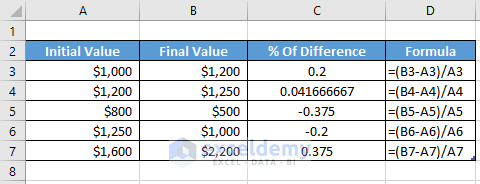Look into the above picture. Here although we applied the percentage of difference formula, the result is not in the percentage format. To get the result in percentage format, select the cells where you want to apply the formatting. After selecting the cells, select the Percentage Style Option which is under the Number section in the Home tab.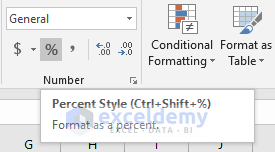After applying the Percentage Style Option, you will get the below result.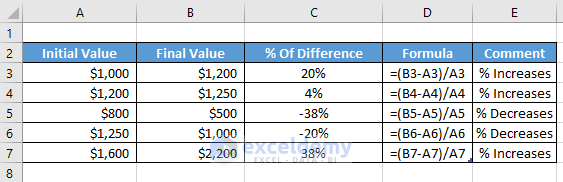## Percentage Difference Between Two Numbers with Custom Number Formatting

Let`s say you want to highlight the percentage of differences such that the positive values get green color and the negative values get the red color. To perform that you need to apply the Custom Number Formatting. First, select the cells where you want to apply this formatting and click on to the right button of your mouse to select the Format Cells option.After selecting the Format Cells option you will get the Format Cells Dialogue box. In the Format Cells dialogue box select the Custom option and, in the Type filed are write, [Green]+ 0%; [Red]- 0%; 0% and press OK.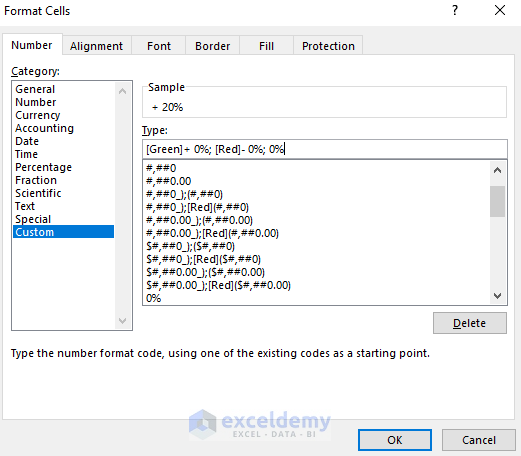After this, you will get to see the below result.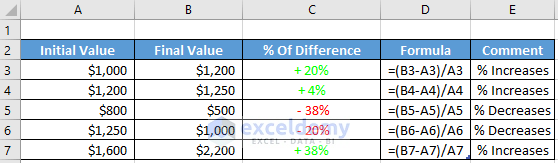## Conclusion

After reading this article, you will learn the excel formula to calculate the percentage difference between the two numbers in Excel. Here I demonstrated two different formatting methods which can be applied to show the result in the percentage format. Feel free to comment if you have any confusions and stay connected to see more useful articles.

Hello!

Welcome to my Profile. Here I will be posting articles related to Microsoft Excel. I have completed my BSc in Electrical and Electronic Engineering from American International University-Bangladesh. I am a diligent, goal-oriented engineer with an immense thirst for knowledge and attitude to grow continuously. Continuous improvement and life-long learning is my motto.

1. ReplyAl Theodoric Feb 8, 2019 at 7:54 PM

So if Microsoft isn’t prepared to fix the DATEDIFF function – what is the alternative method of finding Years and part DAYS between dates.

• ReplyFeb 9, 2019 at 12:36 PM

You can use the DATEDIF Function without any problem.
Thanks.

2. Replyrobotgh0st Jun 28, 2019 at 11:03 PM

Sorry, isn’t this calculating the “percent change” and not the “percent difference”? Percent difference is the difference between two values divided by the average of the two values shown as a percentage.